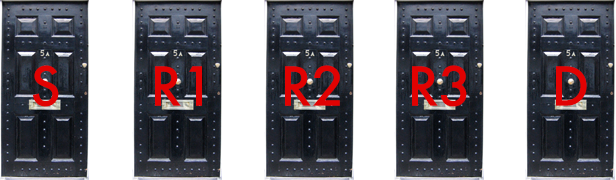# Three Rooms, Safety, and Death

Probability Level 4You have been caught by a gangster who gives you the chance of escaping by playing a simple game. There are 5 rooms, connected in a cycle as $S-R_1-R_2-R_3-D$. You are blindfolded. For each room you go, you must choose one door randomly and enter it.

If you reach $S$ you are released, but if you reach $D$ you are killed. If you start at $R_1$, the probability that you will survive the game is $\frac{p}{q}$, where $p$ and $q$ are coprime positive integers. Find $p+q$.

Note that you have two choices for the door to enter in each room. You can visualize the rooms as vertices of a regular pentagon, which are labeled in order as $S-R_1-R_2-R_3-D.$

×

Problem Loading...

Note Loading...

Set Loading...